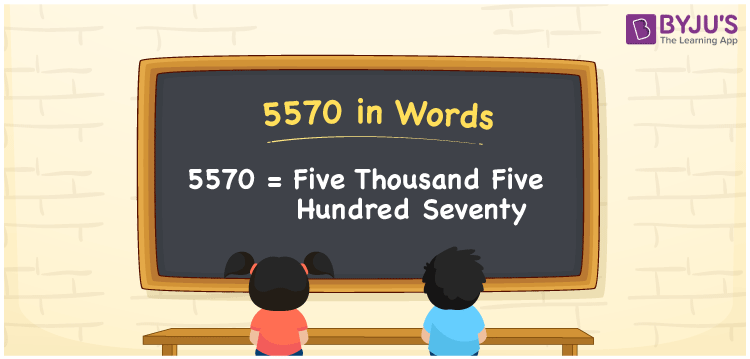# 5570 in Words

The number 5570 in words is written as Five Thousand Five hundred Seventy. The number 5570 is a cardinal number. For example, the cost of 2 shoes is Rs. 5570. In this article, we are going to learn the procedure of writing the number 5570 in words using the place value system in detail.

 5570 in Words: Five Thousand Five Hundred Seventy. Five Thousand Five Hundred Seventy in Numerical Form: 5570.

## 5570 in English Words## How to Write 5570 in Words?

The number 5570 has four digits and its place values are presented below:

 Thousands Hundreds Tens Ones 5 5 7 0

The expanded form of 5570 is as follows:

= 5 × Thousand + 5 × Hundred + 7 × Ten + 0 × One

= 5 × 1000 + 5 × 100 + 7 × 10

= 5000 + 500 + 70

= 5570

= five thousand five hundred seventy

Hence, 5570 in words is five thousand five hundred seventy.

5570 in words – Five thousand five hundred seventy

Is 5570 an odd number? – No

Is 5570 an even number? – Yes

Is 5570 a perfect square number? – No

Is 5570 a perfect cube number? – No

Is 5570 a prime number? – No

Is 5570 a composite number? – Yes

## Frequently Asked Questions on 5570 in Words

Q1

### Write 5570 in words.

5570 in words is five thousand five hundred seventy.

Q2

### Simplify 5000 + 570, and express it in words.

Simplifying 5000 + 570, we get 5570. Hence, 5570 in words is five thousand five hundred seventy.

Q3

### Is 5570 a composite number?

Yes, 5570 is a composite number.